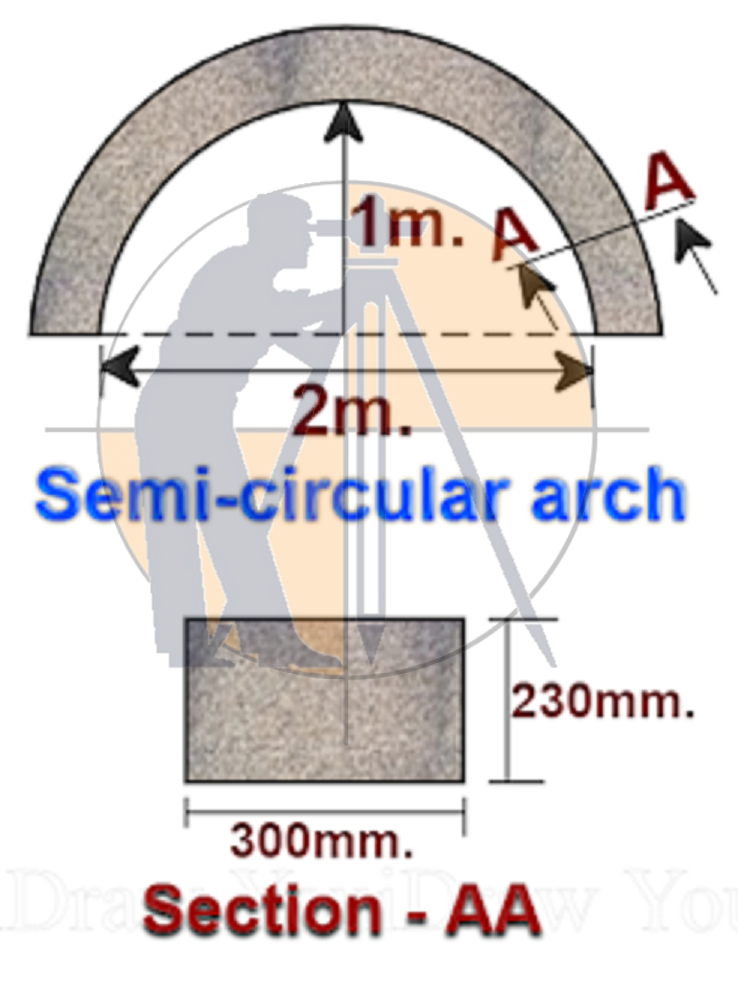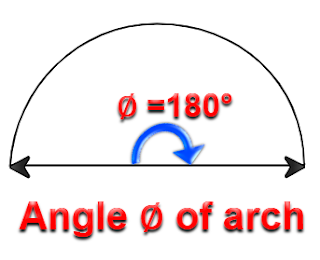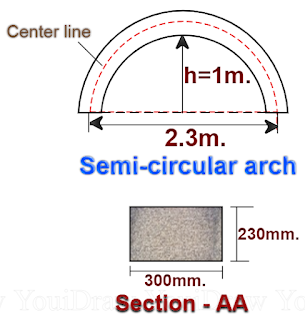Breaking News
Home / Civil Engineering / How To Calculate The length And volume Of Semi Circular Arch

# How To Calculate The length And volume Of Semi Circular Arch

## How To Calculate The length And volume Of Semi Circular Arch

#### Let us now calculate the length & volume of the semi-circular arch as shown below.### Here

#### ∅ = angle formed by the arch as shown below.#### Calculate the volume of the arch, let us calculate the radius of the center line as shown below.## . Land Surveying & Architects

### THANKS.